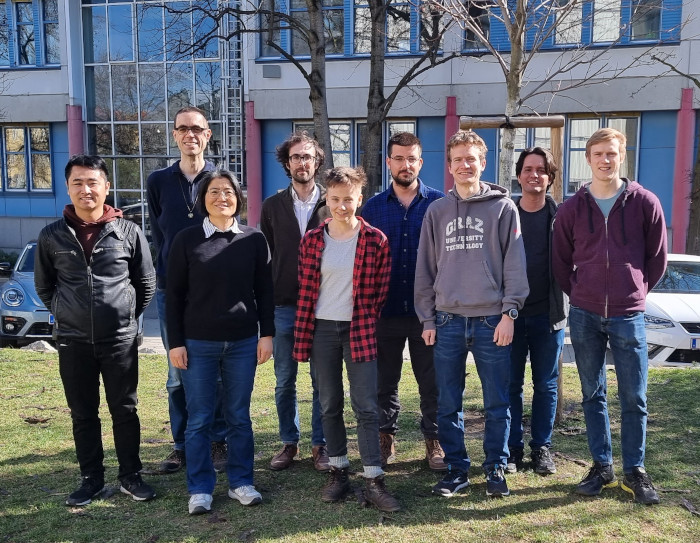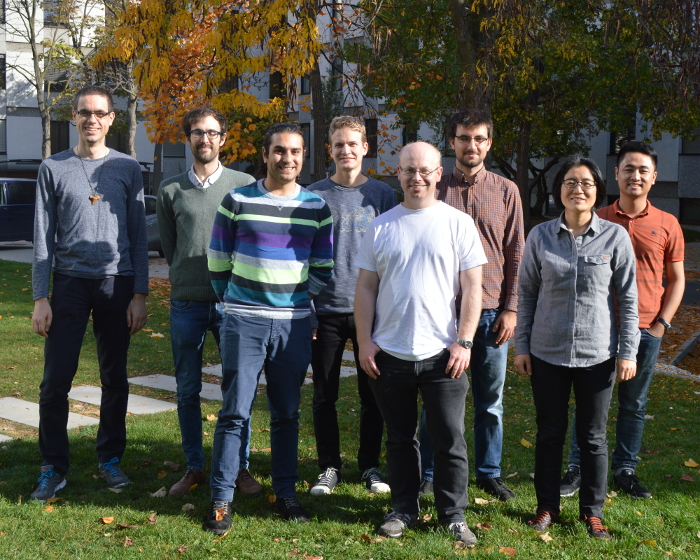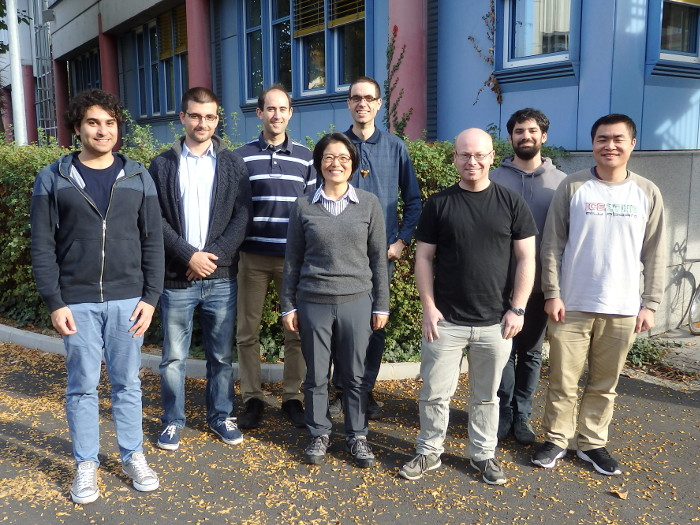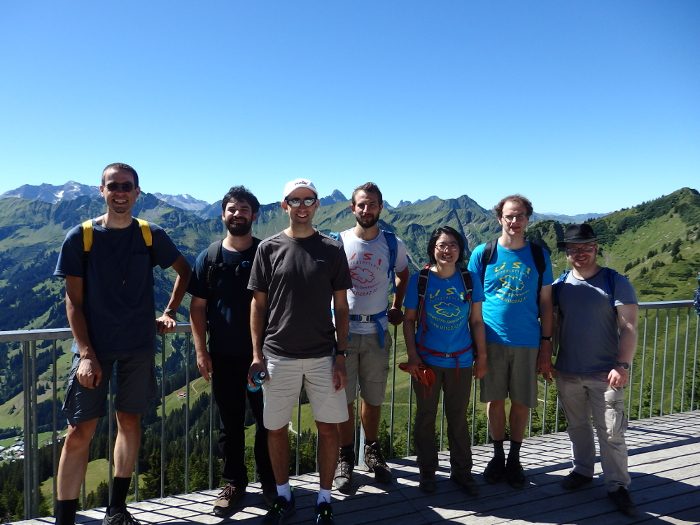Welcome to the Combinatorics Group at TU Graz!

The research of the Combinatorics Group focuses on problems in Combinatorics, Graph Theory, Discrete Probability, and Theoretical Computer Science. The research fields of members of the Combinatorics Group include

• Random graphs, random hypergraphs, random simplicial complexes, random graphs on surfaces
• Structural, enumerative, and algorithmic aspects of graphs
• Randomised algorithms, analysis of algorithms

The Combinatorics Group established in 2012 at the former Institute of Optimization and Discrete Mathematics is part of the Institute of Discrete Mathematics since 2016.

It takes an active part in the Doctoral School of Mathematics and Scientific Computing and the Doctoral Program "Discrete Mathematics" (Project 15 "Random graphs on a surface").The Combinatorics Group in March 2023The Combinatorics Group in October 2019The Combinatorics Group in October 2018The Combinatorics Group in August 2016